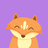• matlabrem函数
千次阅读
2021-04-18 05:35:51

rem(x,y):求整除x/y的余数

mod(x,y):求模

rem(x,y)=x-y.*fix(x./y); (fix()向0取整)

mod(x,y)=x-y.*floor(x./y); (floor()向左取整)

如果x和y的符号相同(同为‘+’，同为‘-’)，那么rem(x,y)=mod(x,y)(正数与正数，负数与负数，取整结果两个函数效果一样)

如果x和y的符号相反，那么mod(x,y)=rem(x,y)+y(正数与负数的取整，看你希望得到什么结果就选择用什么函数)

当正数与负数取余时，当得到的余数结果的符号希望跟除数(x)一样，用rem()函数；当得到的余数结果的符号希望跟被除数(y)一样，用mod()函数

例如：

rem(3,2)=1;mod(3,2)=1;

rem(-3,-2)=-1;mod(-3,-2)=-1;

rem(3,-2)=1;mod(3,-2)=-1;

rem(-3,2)=-1;mod(-3,2)=1;

mod(3,2)=rem(3,2)=1;

mod(3,-2)=rem(3,-2)-2=1-2=-1;

mod(-3,2)=rem(-3,2)+2=-1+2=1;

更多相关内容
• ## Matlab中rem函数使用

万次阅读 多人点赞 2020-12-07 13:22:09
rem函数是两个数相除之后的余数。 一.语法 r = rem(a,b) 1.输入参数 a-被除数 被除数，指定为标量、向量、矩阵或多维数组。a必须是任意数值类型的实数值数组。数值输入a和b必须具有相同的大小或兼容的大小...

目录

语法

说明

示例

标量被除后的余数

向量被除后的余数

正值和负值被除后的余数

浮点值被除后的余数

rem函数是两个数相除之后的余数。

## 语法

a - 被除数

被除数，指定为标量、向量、矩阵或多维数组。a 必须是任意数值类型的实数值数组。数值输入 a 和 b 必须具有相同的大小或兼容的大小（例如，a 是 M×N 矩阵，b 是标量或 1×N 行向量）。

如果 a 和 b 是持续时间数组，则它们必须具有相同的大小，除非其中一个为标量。如果一个输入是持续时间数组，则另一个输入可以是持续时间数组或数值数组。在此上下文中，rem 将数值视为标准 24 小时制的数字。

如果一个输入是整数数据类型，则另一输入必须是相同的整数类型或 double 标量。

b - 除数

除数，指定为标量、向量、矩阵或多维数组。b 必须是任意数值类型的实数值数组。数值输入 a 和 b 必须具有相同的大小或兼容的大小（例如，a 是 M×N 矩阵，b 是标量或 1×N 行向量）。可以参考基本运算的兼容数组大小。

如果 a 和 b 是持续时间数组，则它们必须具有相同的大小，除非其中一个为标量。如果一个输入是持续时间数组，则另一个输入可以是持续时间数组或数值数组。在此上下文中，rem 将数值视为标准24小时制的数字。

如果一个输入是整数数据类型，则另一输入必须是相同的整数类型或 double 标量。

## 说明

r = rem(a,b) 返回 a 除以 b 后的余数，其中 a 是被除数，b 是除数。此函数通常称为求余运算，表达式为 r = a - b.*fix(a./b)。rem 函数遵从 rem(a,0) 是 NaN 的约定。

## 示例

### 标量被除后的余数

计算 23 除以 5 后的余数。

a = 23;
b = 5;
r = rem(a,b)

r = 3

### 向量被除后的余数

计算一个整数向量除以除数 3 的余数。

a = 1:5;
b = 3;
r = rem(a,b)

r = 1×5

1     2     0     1     2


### 正值和负值被除后的余数

计算一个含正负值的整数集被除后的余数。请注意，非零结果的符号与被除数相同。

a = [-4 -1 7 9];
b = 3;
r = rem(a,b)

r = 1×4

-1    -1     1     0

### 浮点值被除后的余数

计算多个角除以除数 2*pi 后的余数。rem 将尝试补偿浮点四舍五入取整的影响，以尽可能生成精确的整数结果。

theta = [0.0 3.5 5.9 6.2 9.0 4*pi];
b = 2*pi;
r = rem(theta,b)

r = 1×6

0    3.5000    5.9000    6.2000    2.7168         0

展开全文• ## 【 MATLAB 】rem函数介绍

万次阅读 多人点赞 2018-10-29 14:13:27
rem函数和mod函数很相似，二者认真看一个，另一个看一下区别即可。 mod函数介绍：【 MATLAB 】mod 函数介绍 rem Remainder after division Syntax r = rem(a,b) Description r = rem(a,b) returns the ...

rem函数和mod函数很相似，二者认真看一个，另一个看一下区别即可。

mod函数介绍：【 MATLAB 】mod 函数介绍

# rem

Remainder after division

## Syntax

r = rem(a,b)

## Description

r = rem(a,b) returns the remainder after division of a by b, where a is the dividend and b is the divisor. This function is often called the remainder operation, which can be expressed asr = a - b.*fix(a./b). The rem function follows the convention that rem(a,0) is NaN.

a/b之后的余数便是r。如果除数为0，则rem(a,0)为NaN，这和我们的认知也很符合。

### Remainder After Division of Scalar

Compute the remainder after dividing 5 into 23.

a = 23;
b = 5;
r = rem(a,b)
r = 3

### Remainder After Division of Vector

Find the remainder after division for a vector of integers and the divisor 3.

a = 1:5;
b = 3;
r = rem(a,b)
r = 1×5

1     2     0     1     2


### Remainder After Division for Positive and Negative Values

Find the remainder after division for a set of integers including both positive and negative values. Note that nonzero results have the same sign as the dividend.

a = [-4 -1 7 9];
b = 3;
r = rem(a,b)
r = 1×4

-1    -1     1     0

注：从这个例子开始，就和mod函数不一样了，这里余数符号与被除数一致。

a = [-4 -1 7 9];
b = 3;
r1 = rem(a,b)
r2 = mod(a,b)

r1 =

-1    -1     1     0

r2 =

2     2     1     0

二者结果是不一样的，我们想想这个rem是怎么计算的。

它是先将被输出a中的符号去掉，当成正数来算，结果符号在和a一致即可。

### Remainder After Division for Floating-Point Values

Find the remainder after division for several angles using a divisor of 2*pi. When possible, rem attempts to produce exact integer results by compensating for floating-point round-off effects.

theta = [0.0 3.5 5.9 6.2 9.0 4*pi];
b = 2*pi;
r = rem(theta,b)
r = 1×6

0    3.5000    5.9000    6.2000    2.7168         0

### Differences Between mod and rem

The concept of remainder after division is not uniquely defined, and the two functions mod and rem each compute a different variation. The mod function produces a result that is either zero or has the same sign as the divisor. The rem function produces a result that is either zero or has the same sign as the dividend.

Another difference is the convention when the divisor is zero. The mod function follows the convention that mod(a,0) returns a, whereas the rem function follows the convention that rem(a,0)returns NaN.

Both variants have their uses. For example, in signal processing, the mod function is useful in the context of periodic signals because its output is periodic (with period equal to the divisor).

展开全文• 满意答案libaoxin2008推荐于 2019.10.24matlabrem()和mod()函数(2010-11-16 13:39:55) 转载rem(x,y):求整除x/y的余数mod(x,y):求模rem(x,y)=x-y.*fix(x./y); (fix()向0取整)mod(x,y)=x-y.*floor(x./y); (floor()向...

满意答案libaoxin2008

推荐于 2019.10.24

matlab的rem()和mod()函数(2010-11-16 13:39:55) 转载

rem(x,y):求整除x/y的余数

mod(x,y):求模

rem(x,y)=x-y.*fix(x./y); (fix()向0取整)

mod(x,y)=x-y.*floor(x./y); (floor()向左取整)

如果x和y的符号相同(同为‘+’，同为‘-’)，那么rem(x,y)=mod(x,y)(正数与正数，负数与负数，取整结果两个函数效果一样)

如果x和y的符号相反，那么mod(x,y)=rem(x,y)+y(正数与负数的取整，看你希望得到什么结果就选择用什么函数)

当正数与负数取余时，当得到的余数结果的符号希望跟除数(x)一样，用rem()函数；当得到的余数结果的符号希望跟被除数(y)一样，用mod()函数

例如：

rem(3,2)=1;mod(3,2)=1;

rem(-3,-2)=-1;mod(-3,-2)=-1;

rem(3,-2)=1;mod(3,-2)=-1;

rem(-3,2)=-1;mod(-3,2)=1;

mod(3,2)=rem(3,2)=1;

mod(3,-2)=rem(3,-2)-2=1-2=-1;

mod(-3,2)=rem(-3,2)+2=-1+2=1;

00分享举报

展开全文• %求整数x/y的余数 rem(x,y) %求模 mod(x,y) 如果x和y的符号相同（同为...当正数与负数取余时，当得到的余数结果的符号希望跟除数(x)一样，用rem()函数；当得到的余数结果的符号希望跟被除数(y)一样，用mod()函数 ...开发语言
• matlab函数大全-matlab函数大全.doc 比较白痴的东西，不过对初学者也许有用，特分享一下。不要喷我哦!函数具体用法可以用help查一下。附件内容与下面一样的。 Aabs 绝对值、模、字符的ASCII码值 acos 反余弦 ...
• mod函数用于取模运算。 语法形式为 M = mod(x,y)，这里的x,y可以是标量|向量|矩阵|多维数组。 M = mod(x,y)返回用 y除以 x后的余数，其中 x是被除数，y是除数。 计算方式为：当 y≠0 时，M=mod(x,y)= x - y.*...
• 转：https://blog.csdn.net/panghaomingme/article/details/70308290
• rem(x,y):求整除x/y的余数mod(x... (floor()向左取整)如果x和y的符号相同(同为‘+’，同为‘-’)，那么rem(x,y)=mod(x,y)(正数与正数，负数与负数，取整结果两个函数效果一样)如果x和y的符号相反，那么mod(x,y)=rem(...
• matlab_函数大全 数组和矩阵(基本信息)回目录 disp 显示文本或数组 display 显示文字或数组（重载方法） isempty 确定是否为空数组 isequal 测试数组平等 isequalwithequalnans 测试数组平等，平等对待纳斯 isfinite...
• matlab常用函数-常用函数表.doc matlab常用函数
• MATLAB常用函数-MATLAB常用函数.doc 希望能给初用MATLAB的朋友帮助
• MATLAB函数库-MATLAB函数库.doc MATLAB函数
• ## matlab中rem与mod函数的区别

万次阅读 多人点赞 2015-10-10 08:50:53
rem(x,y):求整除x/y的余数 mod(x,y):求模   rem(x,y)=x-y.*fix(x./y); (fix()向0取整) mod(x,y)=x-y.*floor(x./y); （floor()向左取整,以数抽为准，朝负无穷方向取整） 如果x和y的符号相同（同为...
• ## matlab基本函数

千次阅读 2021-04-05 09:41:04
*matlab基本函数 1.生成矩阵 （1）直接法：a = [1,2,3;4,5,6;7,8,9] （2）冒号一维矩阵： a = 开始：步长：结束，步长为1可省略 b = 1:1:10; b = 1:10; （与上面等价） （3）函数生成： linspace(开始，结束，...
• 目录 1、求组合数 2、求阶乘 3、求全排列 4、求指数 5、求行列式 6、求矩阵的转置 7、求向量的指数 ...20、常用的基本数学函数 ...21、常用的三角函数 ...22、适用于向量的常用函数 ...23、MATLAB的永久常数 24、
• matlab常用指令和函数.doc常用指令和函数
• Matlab自定义函数的七种方法1、函数文件+调用命令文件：需单独定义一个自定义函数的M文件。2、函数文件+子函数：定义一个具有多个自定义函数的M文件。3、Inline:无需M文件，直接定义。4、匿名函数。5、Syms+subs:...
• ## matlab常用函数

千次阅读 多人点赞 2019-09-07 16:33:57
• 虽然matlab中提供了几个随机数函数，但一些情况下（如需要在-1到1中产生随机数)用matlab中自带的随机函数无法直接达到想要的效果。那么我们不如自己写一个函数出来用，在matlab中我们只要创建一个和函数名一样的m...
• ## Matlab常用函数和命令大全

千次阅读 多人点赞 2021-02-07 11:59:33
path 设置或查询Matlab路径 附录1.2管理变量与工作空间用命令 函数名 功能描述 函数名 功能描述 clear 删除内存中的变量与函数 pack 整理工作空间内存 disp 显示矩阵与文本 save 将工作空间中的变量存盘 ...
• Matlab作为一种强大的处理软件，里面的很多函数对初学者来说是一个较大的障碍，现将相关函数总结整理供大家参考学习
• 常用函数Matlab内部常数eps：浮点相对精度exp：自然对数的底数ei 或 j：基本虚数单位inf 或Inf：无限大，例如1/0nan或NaN：非数值(Not a number)，例如0/0 pi：圆周率 p(= 3.1415926...)realmax：系统所能表示的...
• ## Matlab函数参数汇总

千次阅读 2021-04-23 18:15:38
Matlab 函数参数汇总Saturday, October 27, 2007 12:49:05 AMMATLAB函数参考附录1.1 管理用命令函数名 功能描述 函数名 功能描述addpath 增加一条搜索路径 rmpath 删除一条搜索路径demo 运行Matlab演示程序 type 列...
• 目录 1.随机数产生类 2.数学函数类 3.做图类函数 4.信号处理类 5.其他 ...函数：randn： 产生标准正态随机变量 ...2.数学类函数 ...Matlab函数名：acos（x）：反余弦函数 acot（x）：反余切函数 ...
• Matlab函数文件prime.m的用法1.函数文件prime.mfunction prime(a)if size(a)~=1|round(a)~=a %判断输入的变量是否为一个整数标量error('The input argument must be a interger number')endfor i=2:sqrt(a) %判断a...
• matlab常用函数与常用指令大全 matlab常用函数- - 1、特殊变量与常数 ans 计算结果的变量名 computer 确定运行的计算机 eps 浮点相对精度 Inf 无穷大 I 虚数单位 inputname 输入参数名 NaN 非数 nargin 输入参数个数...
• 五、数组和矩阵函数 1）数组基本函数 display：显示字符或者数组 isempty :判断数组是否为空，空返回1，不空返回0 isequal :判断数组是否相同 （认为NaN不同） isequalwithequalnans:判断数组是否相同，把NaN......

# matlabrem函数matlab 订阅